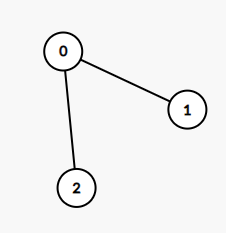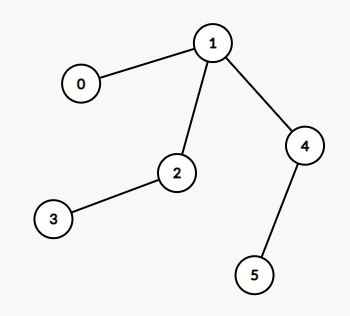1245. Tree Diameter

Given an undirected tree, return its diameter: the number of edges in a longest path in that tree.

The tree is given as an array of `edges` where `edges[i] = [u, v]` is a bidirectional edge between nodes `u` and `v`.  Each node has labels in the set `{0, 1, ..., edges.length}`.

Example 1:```Input: edges = [[0,1],[0,2]]
Output: 2
Explanation:
A longest path of the tree is the path 1 - 0 - 2.
```

Example 2:```Input: edges = [[0,1],[1,2],[2,3],[1,4],[4,5]]
Output: 4
Explanation:
A longest path of the tree is the path 3 - 2 - 1 - 4 - 5.
```

Constraints:

• `0 <= edges.length < 10^4`
• `edges[i] != edges[i]`
• `0 <= edges[i][j] <= edges.length`
• The given edges form an undirected tree.

1245. Tree Diameter
``````struct Solution;

impl Solution {
fn tree_diameter(edges: Vec<Vec<i32>>) -> i32 {
let n = edges.len() + 1;
let mut graph = vec![vec![]; n];
for e in edges {
let u = e as usize;
let v = e as usize;
graph[u].push(v);
graph[v].push(u);
}
let mut visited = vec![false; n];
let mut res = 0;
Self::dfs(0, &mut visited, &mut res, &graph);
res as i32
}

fn dfs(u: usize, visited: &mut Vec<bool>, diameter: &mut usize, graph: &[Vec<usize>]) -> usize {
visited[u] = true;
let mut max_depth = 0;
for &v in &graph[u] {
if !visited[v] {
let depth = Self::dfs(v, visited, diameter, graph);
*diameter = (*diameter).max(depth + max_depth);
max_depth = max_depth.max(depth);
}
}
max_depth + 1
}
}

#[test]
fn test() {
let edges = vec_vec_i32![[0, 1], [0, 2]];
let res = 2;
assert_eq!(Solution::tree_diameter(edges), res);
let edges = vec_vec_i32![[0, 1], [1, 2], [2, 3], [1, 4], [4, 5]];
let res = 4;
assert_eq!(Solution::tree_diameter(edges), res);
}
``````